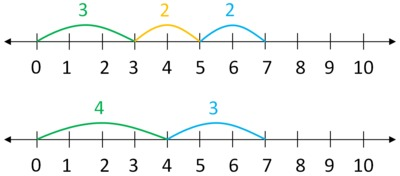# Frog and Toad on the number line

Alignments to Content Standards: 2.MD.B.6

One day, Frog and Toad were sitting together on a lily pad. Some lily pads were in a line across the pond.In the morning, Frog hopped three lily pads away. In the afternoon, he hopped two more away. In the evening, he hopped another two more.

Toad hopped four lily pads away in the morning. He rested in the afternoon and continued three further in the evening. Frog said,

Toad, we ended up at the same place!

Show each of their journeys on a number line, starting at $0$. Use different colors for the morning, afternoon, and evening hops. Write a number sentence that reflects that they ended up at the same place.

## IM Commentary

The purpose of this task is for students to use a number line to show why two different sums are equal and to represent that equality with an equation. This task could be used to introduce the idea of representing addition on the number line; the teacher could set up a number line on the board or floor so students can act out where the two amphibious friends end up.

Students who have only written equations of the form $A + B = C$ may not realize that we can write equations that show two sums are equal without actually writing the value of the sum. Students may also be unfamiliar with writing equations with more than two addends on a side. If this is the case, then the teacher might need to write the equation the first time they see a problem like this. For some students the teacher may also need to scaffold this concept by showing $3 + 2 + 2 = 7$ and $4 + 3 = 7$. Once students have seen that an equation like $3 + 2 + 2 = 4 + 3$ is an appropriate response, they are much more likely to produce such equations on their own.

The Standards for Mathematical Practice focus on the nature of the learning experiences by attending to the thinking processes and habits of mind that students need to develop in order to attain a deep and flexible understanding of mathematics. Certain tasks lend themselves to the demonstration of specific practices by students. The practices that are observable during exploration of a task depend on how instruction unfolds in the classroom. While it is possible that tasks may be connected to several practices, only one practice connection will be discussed in depth. Possible secondary practice connections may be discussed but not in the same degree of detail.

This particular task helps illustrate Mathematical Practice Standard 2, Reason abstractly and quantitatively. Students make sense of quantities and how they are related in a problem situation. In the task at hand, students first create meaningful representations of the problems (3 jumps + 2 jumps + 2 Jumps and 4 jumps + 3 jumps) by using number lines. Then, they write equations for each and realize that the two sums are equal and that they can write the two expressions as equal to each other. During this process, students periodically contextualize the problem by connecting the mathematical symbols back to the context. Thus, students build meaning for the mathematical symbols by reasoning about the problem rather than memorizing an abstract set of rules or procedures. Problems that begin with a context and are represented with mathematical objects or symbols can also be examples of modeling with mathematics (MP.4).

## Solution

Frog's journey is shown on the top number line and Toad's journey is shown on the bottom number line:The number sentence $$\Large{3+2+2=4+3}$$ shows that Frog and Toad ended up at the same place.